# Introduction

#### Acids and Bases: Introduction

A solution is defined as a homogeneous mixture of two substances, the solute and the solvent. The solute is defined either as the substance that changes state or the substance present in the smallest amount in the solution. The solvent is defined as either the substance that does not change its state or the substance present in the largest amount in the solution. The simplest example is a solution of sugar water, prepared by dissolving a small amount of table sugar, the solute, in water, the solvent. The sugar changes state (solid to liquid) and is present in the smallest amount. Solutions most commonly consist of a solid solute and a liquid solvent but the solute and solvent can be in the gaseous, liquid, or solid state.

Table sugar, like some chemical compounds, is not changed chemically when dissolved in a solution. The molecules of sugar are the same whether the sugar is a solid or dissolved in the solution. Other chemical compounds, when dissolved in a solution, dissociate or separate into two or more different chemical compounds called ions. Cations are ions that are positively charged and anions are ions that are negatively charged. An example of a solute that dissociates is table salt or sodium chloride. When sodium chloride, NaCl, is dissolved in water, it dissociates into the cation Na+and the anion Cl.

The amount of solute dissolved in a solvent is quantified by its concentration, defined as the ratio of the amount of solute to the volume of solvent. Concentration can be expressed using different units depending on the context. Grams of solute per milliliter, per 100ml or per liter are common units. Concentration can be expressed as a weight/volume percentage, such as a 10% salt solution, in which the concentration is expressed as grams of solute per 100ml of solvent. Therefore, a 10% salt solution contains 10g of salt for every 100ml of solution. Concentration can also be expressed as a volume/volume percentage as in the 70% isopropyl alcohol in rubbing alcohol. This concentration is expressed as a solution of 30ml of water, the solute, and 70ml of isopropyl alcohol, the solvent.

In chemistry, concentration is often expressed as a molar concentration or moles per liter using a capital letter M as the unit designation. This, in effect, expresses the number of molecules of solute dissolved per liter. If a solute dissociates into different numbers of ions, then the concentration is expressed as a normal concentration using a capital letter N as the unit designation. A 1 Molar solution of NaCl contains 1 mole of both Na+ and Cl. A 1 Molar solution of MgCl2 contains 1 mole of Mg+ but 2 moles of Cl. For this reason, this solution would be a 1 Normal (or 1 Molar) solution of Mg+ ion and a 2 Normal solution of Cl ion.

Regardless of which units are used, the concentration of any solute can be increased simply by adding more solute. The concentration of a solute can be decreased by adding more solvent to dilute the original solution. For example, a chemist has 1 liter of a 100g/liter solution but requires a 50g/liter solution. They can simply add an additional liter of solvent to the original solution and prepare 2 liters of the required 50g/liter concentration.

A dilution like the one described above is not possible if the desired concentration is significantly more dilute than the original solution. For example, if the chemist above requires a 0.01g/liter solution, they would need to add an additional 9,999 liters of solvent to the original liter of solution to dilute it to a 0.01g/liter concentration. Alternatively, they could add 0.1ml of the original 100g/liter solution to 1 liter of solvent. Clearly, neither method is very convenient since the chemist must either add a very large volume of solvent or measure a very small volume of the original solution. A dilution technique, called serial dilution, must be used in which a series of dilutions is used to prepare progressively lower concentrations of solution until the desired concentration is reached. The following diagram illustrates the example.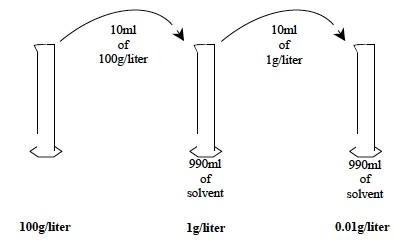A 10ml volume of the original 100g/liter solution is added to 990ml of solvent in the second graduated cylinder and mixed to produce a concentration of 1g/liter or 1/100th the original concentration. A 10ml volume of the 1g/liter solution is added to 990ml in another graduated cylinder and mixed to produce a concentration of 0.01g/liter or 1/100th the concentration of the 1g/liter solution. The series of dilutions produce solutions of intermediate concentrations that can then be diluted to produce a solution whose concentration is much significantly dilute than the original solution.

Acids are a group of covalent compounds that, when in solution in water, dissociate into one or more hydrogen ions (H+), and a negatively charged ion. Consider the example of hydrochloric acid, HCl, the acid that aids digestion in the stomach. When HCl is dissolved in water, it dissociates into the cation H+ and the anion Claccording to the following equation: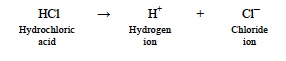Since the hydrogen ion (H+) is very reactive, the chemical properties of acids are determined by the H+ ion concentration. The concentration of H+ ions is, in turn, determined by the amount of dissolved acid and by the extent of dissociation. Strong acids, like HCl, dissociate completely resulting in a high H+ ion concentration. Weak acids, like the acetic acid in vinegar, do not dissociate completely resulting in a low H+ ion concentration. In this Core Experience Learning Lab, however, differences in dissociation among acids will not be addressed. Differences among acids will be referred to only as differences due to whether the acid is strong or weak.

Bases are ionic compounds that, like acids, also dissociate when dissolved in water. Consider the example of sodium hydroxide, NaOH, the base found in drain cleaner. When NaOH is dissolved in water, it dissociates into the hydroxide anion, OH, and the cation Na+ according to the following equation: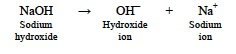Note: Alcohols such as rubbing alcohol also contain OH groups. However, alcohols are not bases since they are covalent, organic compounds and not ionic compounds like bases.

Notice that the dissociation reaction of bases does not directly involve hydrogen ions. However, when both a base and an acid are dissolved in water, a neutralization reaction takes place that reduces the hydrogen ion concentration. In water solution, both a base and an acid will dissociate as shown in the previous two equations. When this occurs, the OH and H+ ions react to form water, H2O, according to the following equation: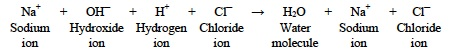Notice that the net result of a neutralization reaction is the production of a water molecule(s), the production of a salt, and a decrease in the hydrogen ion concentration. The reactivity of bases is due to the fact that the hydroxide ion, OH, is very reactive and reacts readily with H+ ions.

The hydrogen ion concentrations of typical solutions are quite low, ranging from 1 to 0.00000000000001 moles per liter (M). When these concentrations are expressed using either decimals or scientific notation (100 to 10-14) the very small decimals or negative exponents are difficult to read and interpret. A simplified scale for expressing the hydrogen ion concentration is the pH Scale. The pH Scale simply expresses the hydrogen ion concentration as the positive exponent of the hydrogen ion. For example, a hydrogen ion concentration of 0.001M or 10-3 M has a pH of 3. A hydrogen ion concentration of 0.00000000001M or 10-11 M has a pH of 11.

Note: More exactly, a molar concentration is converted to pH by taking the negative log of the H+ ion concentration. For example, a molar concentration of 2 x 10-5, is converted to pH by the following method: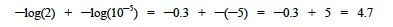In this Core Experience Learning Lab, concentrations will include only powers of 10.

Water is considered both an acid and a base since it can produce both hydrogen ions (H+) and hydroxide ions (OH) in low concentrations according to the following equation: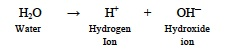Notice that one water molecule produces one hydrogen ion (H+) and one hydroxide ion (OH). In pure water, the concentrations of hydrogen ions (H+) and hydroxide ions (OH) are equal and the pH is 7.

A pH of 7 is considered neutral since the H+ ion concentration of pure water is 10-7moles/liter. Even clean drinking water, however, does not have a neutral pH since it usually contains harmless dissolved chemicals that affect the pH. Even a slight amount of dissolved carbon dioxide gas will lower the pH. A pH of less than 7 (high H+ ion concentration) is considered acidic and a pH of greater than 7 (low H+ ion concentration) is considered basic.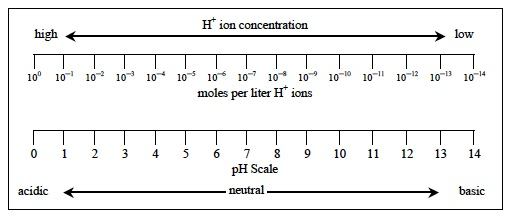Figure 2: Relationship of the hydrogen ion concentration (moles per liter) and the pH Scale.

The pH is easily measured using pH paper strips. These strips contain chemical compounds or pH indicators, which change color in response to differing H+ ion concentrations. These compounds can acquire H+ ions and incorporate them into their chemical structures. This results in a change in their absorption spectra and a change in the perceived color of the compounds depending on the Hion concentration.

#### LEARN THE LABLEARNER LINGO

The following list includes Key Terms that are introduced within the Backgrounds of the CELL. These terms should be used, as appropriate, by teachers and students during everyday classroom discourse.

Note: Additional words may be bolded within the Background(s). These words are not Key Terms and are strictly emphasized for exposure at this time.

##### Investigation 1:
• Acid: a chemical compound that dissociates into a hydrogen ion(s) and a negatively charged ion when mixed with water
• Ion: a chemical compound that has a negative or a positive charge – A cation is positively charged and an anion is negatively charged.
• Hydrogen ion: When an acid is mixed with water, it dissociates into a negatively charged ion and a hydrogen ion, H+. A hydrogen ion is a hydrogen atom that has lost 1 electron, so only the nucleus and its single proton remain.
• pH scale: a linear scale from 0 to 14 that is a measure of the hydrogen ion concentration
• Concentration:  the ratio of the amount of solute to the volume of solvent – Concentration is usually expressed as a certain mass or volume of solute that is dissolved in 1 milliliter, 100 milliliters or in 1 liter.
• Hydroxide ion: When a base is mixed with water, it dissociates into a positively charged ion and a hydroxide ion, OH. A hydroxide ion is a water molecule that has lost a hydrogen ion, H+, leaving its electron.
• Base: a chemical compound that dissociates into hydroxide ion(s) and a positively charged ion when mixed with water
• Dissociate: when a chemical compound is dissolved in a solvent, usually water, and separates into two or more ions

#### Investigation 2:

• Solute: the substance that changes state when a solution is prepared or is present in the smallest amount
• Solvent: the substance that does not change state when a solution is prepared or is present in the largest amount – In the context of these Investigations, the solvent is water.
• Solution: a mixture of two or more substances that is homogeneous or the same throughout
• Dilution: when a less concentrated solution is prepared by adding additional solvent

#### Investigation 3:

• Neutralization: when an acid and a base react to form a water molecule(s) and reduce the hydrogen ion concentration

# CONTENT

• Fun Facts
• Learn the Lingo
• Get Focused

___________________________

## How Antacids Work

Almost everyone has experienced sore stomach pain. Among the most common cause of stomach pain is excess acid in the stomach.As a component of the digestive process, the stomach secrets hydrochloric acid (HCl). This acid helps digest food. However, sometimes the stomach overproduces HCl, which can cause irritation and pain. Antacids are medications taken orally that react with excess stomach acid and “neutralize” it. This often relieves the symptoms of acid stomach.

While there are many different antacids that differ in color, taste, and form (liquids, gums, tablets, etc.), many contain one or more bases such as Al(OH)3  (aluminum hydroxide) or Mg(OH)2 (magnesium hydroxide). When such hydroxide bases react with excess HCl (hydrochloric acid) in the stomach, the following reaction occurs (magnesium hydroxide is used in this example):This reaction “neutralizes” the excess stomach HCl. The reaction results in the formation of the harmless salt, magnesium chloride (MgCl2), and water.

## Logarithms

In this CELL, you will learn that the term pH, which is the measure scientists use to express the acidity of a solution, is the negative log of the hydrogen ion [H+] concentration. But what is a log? The logarithm, abbreviated log, is mathematically related to exponents. Whereas an exponent is written as 103 and indicates multiplying the number 10 three times by itself (10 x 10 x 10 = 1,000), the “natural” log of 1,000 would be 3. In practical terms, this permits us to replace very large or very small numbers in calculations with simple logs. Thus, 1011, which is equal to 100,000,000,000 (one hundred billion or 10 x 10 x 10 x 10 x 10 x 10 x 10 x 10 x 10 x 10 x 10) would simply be written as log 11. This is a very large number.

In nature, there are many examples of how repeating changes in structural characteristics are often related to logarithms. The nautilus shell, for example, increases in size by increasing the volume of the compartments as the animal grows. This increase occurs in a logarithmic fashion.Notice the inner compartments don’t simply double during growth, they increase in size much more rapidly.

Another very beautiful example of logarithms in nature is the arrangement and growth of seeds in the sunflower and other flowers.A quick glance at the sunflower clearly shows the organization of its structure and seed placement in a highly organized, logarithmic manner.

## Logarithms and pH

One of the most important things to realize about natural logarithms is that they do not increase linearly (such as 500 + 500 + 500 + 500 = 2,000), but rather “logarithmically” (10 x 10 x 10 x 10 = 10,000). Thus, in the pH scale that we will be using throughout this CELL and chemists use every day, an increase in pH of 2 gives us a [H+] (hydrogen ion concentration) ten-times higher than a pH of 1. The difference between a linear and logarithmic scale (on the vertical, y-axis) is shown below. The highest number plotted on each of these graphs is 4,250. Notice how, on the logarithmic scale, the data is plotted in a straight line.The most important thing for us to remember for this CELL is that a change in pH from 1 to 2, for example, doesn’t mean that the acidity doubles, but actually increases ten-fold. Thus, if we lowered the pH from 7 to 4, we would have changed the acidity of the solution a thousand-fold!

Finally, the other important point to remember is that we say that pH is the negative log (-log) of the hydrogen ion concentration. In practice, this means that the higher the pH, the less acidic the solution. The lower the pH, the more acidic the solution.

#### GET FOCUSED

The Focus Questions in each Investigation are designed to help teachers and students focus on the important concepts. By the end of the CELL, you should be able to answer the following questions:

##### Investigation 1:
• What properties make acids and bases chemically reactive?
• What is the relationship between the concentration of H+ ions in a solution and the chemical reactivity of strong and weak acids?
• What is the relationship between the concentration of H+ions in a solution and the chemical reactivity of strong and weak bases?
##### Investigation 2:
• What is the relationship between the pH of a solution and the concentration of hydrogen ions (H+)?
##### Investigation 3:
• What happens to the concentration of hydrogen ions (H+) when an acid and a base are reacted?